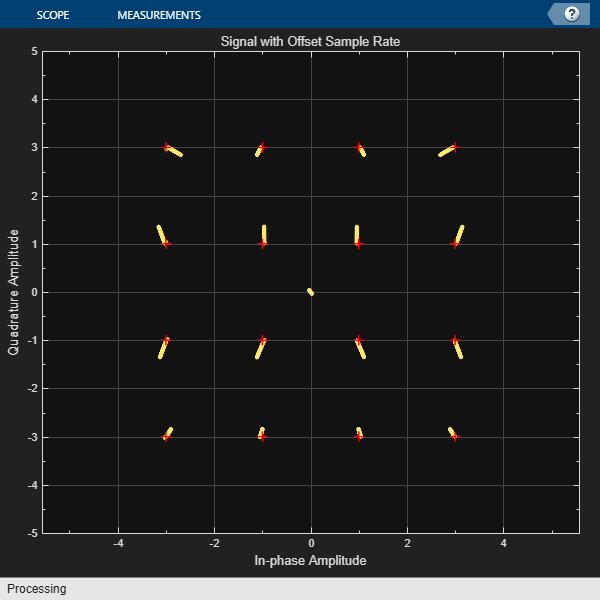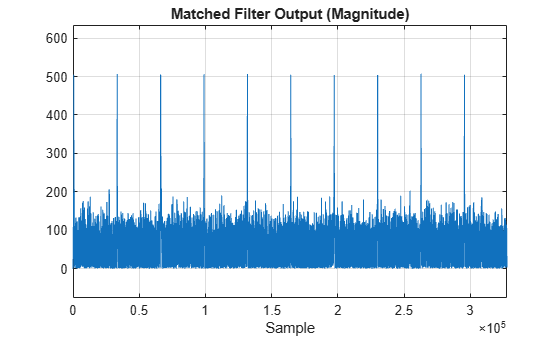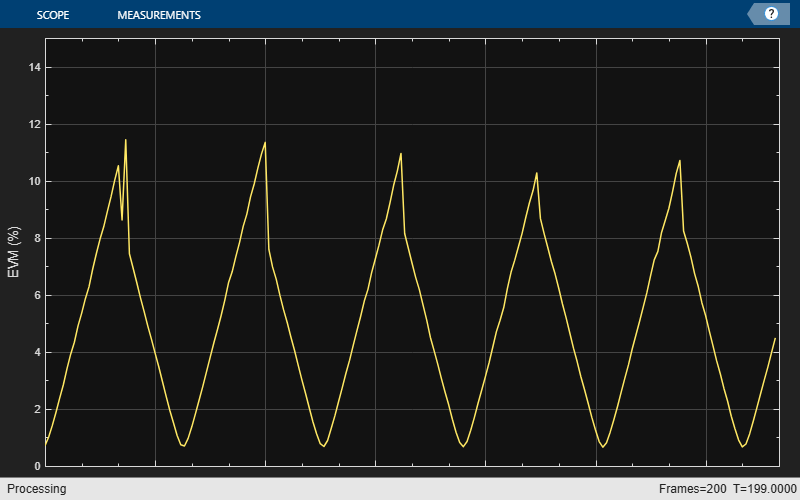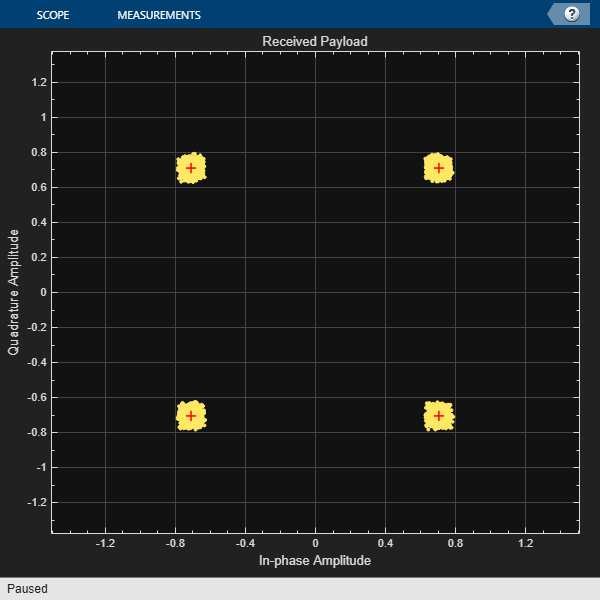# comm.SampleRateOffset

Apply sample rate offset to signal

Since R2021b

## Description

The `comm.SampleRateOffset` System object™ applies a sample rate offset to the input signal. Applying a sample rate offset is equivalent to changing the ADC clock rate.

To apply a sample rate offset to a signal:

1. Create the `comm.SampleRateOffset` object and set its properties.

2. Call the object with arguments, as if it were a function.

## Creation

### Syntax

``sro = comm.SampleRateOffset``
``sro = comm.SampleRateOffset(offset)``
``sro = comm.SampleRateOffset(Offset=offset)``

### Description

````sro = comm.SampleRateOffset` creates a sample rate offset System object.```

example

````sro = comm.SampleRateOffset(offset)` sets the `Offset` property to the value of the `offset` input argument.```
````sro = comm.SampleRateOffset(Offset=offset)` sets the `Offset` property to the value specified by `offset`.```

## Properties

expand all

Sample rate offset in parts per million (ppm), specified as a scalar greater than –1e6.

Data Types: `double`

## Usage

### Syntax

``y = sro(x)``

### Description

example

````y = sro(x)` applies the sample rate offset configured by `sro` to the input signal and returns the resulting signal.```

### Input Arguments

expand all

Input signal, specified as a scalar, an NS element column vector, or an NS-by-NC matrix. NS is the number of time samples. NC is the number of channels. For matrix input signals, the sample rate offset is applied independently to each column.

Data Types: `double` | `single`
Complex Number Support: Yes

### Output Arguments

expand all

Output signal, returned as a scalar, vector or matrix with the same data type as input signal `x`.

## Object Functions

To use an object function, specify the System object as the first input argument. For example, to release system resources of a System object named `obj`, use this syntax:

`release(obj)`

expand all

 `step` Run System object algorithm `release` Release resources and allow changes to System object property values and input characteristics `reset` Reset internal states of System object

## Examples

collapse all

Set parameters and an input signal.

```M = 16; % Modulation order offset = 50; % Parts per million data = (0:M-1)'; % Input signal refconst = qammod(data,M); % Reference constellation points```

Create sample rate offset and constellation diagram System objects.

```sro = comm.SampleRateOffset(offset); constdiagram = comm.ConstellationDiagram( ... 'ReferenceConstellation',refconst, ... 'XLimits',[-5 5], ... 'YLimits',[-5 5], ... 'Title','Signal with Offset Sample Rate');```

Apply 16-QAM modulation to random data, and then apply a sample rate offset to the modulated signal. Plot the reference constellation and the signal with offset sample rate offset.

```modData = qammod(repmat(data,100,1),M); impairedData = sro(modData); constdiagram(impairedData)```Apply sample rate offsets to a 30 kHz single tone sine wave. Confirm the offset by computing the frequency difference between the transmitted tone and the received tone after applying positive and negative sample rate offsets.

Generate a single tone at 30 kHz.

```f = 30e3; samplerate = 100e3; src = dsp.SineWave('Frequency',f, ... 'SampleRate',samplerate, ... 'SamplesPerFrame',10000, ... 'ComplexOutput',true); txsig = src();```

Verify the frequency of the tone.

```freqtx = samplerate * ... (mean(angle(txsig(2:end) ./ txsig(1:end-1)))/(2*pi))```
```freqtx = 3.0000e+04 ```

Apply a sample rate offset of 20 ppm to the transmission tone (`txsig`). Increasing the sample rate offset is equivalent to increasing the ADC clock rate.

```sro = comm.SampleRateOffset(20); rxsig = sro(txsig);```

Find the frequency of the received tone (`rxsig`) after offsetting the sample rate. To skip samples with transient effects, ignore the first 100 samples.

```freqrx = samplerate * ... (mean(angle(rxsig(101:end) ./ rxsig(100:end-1)))/(2*pi))```
```freqrx = 2.9999e+04 ```

Increasing the ADC clock rate, decreases the frequency of the received tone. To show that the frequency of the received tone decreases by approximately 20 ppm, compare the frequency of the transmitted tone to the frequency of the received tone.

`freqchangeppm = (freqrx-freqtx)/freqtx*1e6`
```freqchangeppm = -19.9365 ```

Apply a sample rate offset of -30 ppm to the transmission tone (`txsig`). Decreasing the sample rate offset is equivalent to decreasing the ADC clock rate.

```sro = comm.SampleRateOffset(-30); rxsig = sro(txsig);```

Find the frequency of the received tone (`rxsig`) after offsetting the sample rate. To skip samples with transient effects, ignore the first 100 samples.

```freqrx = samplerate * ... (mean(angle(rxsig(101:end) ./ rxsig(100:end-1)))/(2*pi))```
```freqrx = 3.0001e+04 ```

Decreasing the ADC clock rate, increases the frequency of the received tone. To show that the frequency of the received tone increases by approximately 30 ppm, compare the frequency of the transmitted tone to the frequency of the received tone.

`freqchangeppm = (freqrx-freqtx)/freqtx*1e6`
```freqchangeppm = 30.0736 ```

Display the effects of a sample rate offset in the receiver on a QPSK signal.

Transmit frames containing a fixed preamble and random payload. In the receiver, use the preamble to find the beginning of a frame, and then demodulate the payload and measure the EVM. With a constant nonzero sample rate offset, the EVM varies from frame to frame with a consistent periodicity.

Initialize configuration parameters and create pulse-shaping filter objects for the transmitter and receiver.

```numFrames = 200; numSymbolsPerFrame = 4096; preambleLength = 64; payloadLength = numSymbolsPerFrame - preambleLength; modulationOrder = 4; rolloff = 0.2; filterSpan = 10; samplesPerSymbol = 8; txFilter = comm.RaisedCosineTransmitFilter(RolloffFactor=rolloff, ... FilterSpanInSymbols=filterSpan, ... OutputSamplesPerSymbol=samplesPerSymbol); rxFilter = comm.RaisedCosineReceiveFilter(RolloffFactor=rolloff, ... FilterSpanInSymbols=filterSpan, ... InputSamplesPerSymbol=samplesPerSymbol,DecimationFactor=1);```

Use a Gold sequence for the preamble. Map the Gold sequence to 0.7071 + 0.7071i and -0.7071 -0.7071i in the QPSK constellation.

```goldSeq = comm.GoldSequence(SamplesPerFrame=preambleLength); preamble = goldSeq(); preamble(preamble==1)=2; preambleModOut = pskmod(preamble,modulationOrder,pi/modulationOrder);```

Generate a time-domain reference signal for the preamble.

```preambleRefDelayed = rxFilter( ... txFilter([preambleModOut;zeros(filterSpan,1)])); preambleRef = preambleRefDelayed( ... filterSpan*samplesPerSymbol+(1:samplesPerSymbol*preambleLength));```

Generate a random payload and transmit signal.

```txPayload = pskmod( ... randi([0 modulationOrder-1],payloadLength,numFrames), ... modulationOrder, ... pi/modulationOrder); txFrames = reshape([repmat(preambleModOut,1,numFrames);txPayload],[],1); txSig = txFilter([txFrames;zeros(payloadLength,1)]);```

Apply a 0.8 ppm sample rate offset to the received signal.

```simulatedSRO = 0.8; sro = comm.SampleRateOffset(simulatedSRO); rxSig = rxFilter(sro(txSig));```

Use a matched filter to find the preamble.

```matchedFilterResponse = conj(flipud(preambleRef)); matchedFilterOutMag = abs(filter(matchedFilterResponse,1,rxSig));```

Find the peak locations and plot the first ten peaks.

```threshold = max(matchedFilterOutMag)*0.7; [~, peakLocations] = findpeaks(matchedFilterOutMag, ... MinPeakHeight=threshold, ... MinPeakDistance=preambleLength*samplesPerSymbol); plot(matchedFilterOutMag) title('Matched Filter Output (Magnitude)') xlabel('Sample') axis([0 numSymbolsPerFrame*samplesPerSymbol*10 ... -0.15*max(matchedFilterOutMag) 1.25*max(matchedFilterOutMag)]); grid on```Locate the frames and examine the received data by plotting the computed EVM value and the received signal constellation. Estimate the sample rate offset. For a more accurate estimate, you can increase the number of transmitted frames.

```frameDelay = peakLocations - length(preambleRef); constDiag = comm.ConstellationDiagram(Title='Received Payload', ... Position=[20 70 600 600]); evm = comm.EVM; evmScope = timescope(TimeUnits='none',TimeSpan=length(frameDelay), ... YLabel='EVM (%)',YLimits=[0 15],TimeAxisLabels='none', ... Position=[650 120 800 500]); for i = 1:length(frameDelay) rxFrame = rxSig(frameDelay(i) + ... (1:samplesPerSymbol:numSymbolsPerFrame*samplesPerSymbol)); evmScope(evm(txPayload(:,i),rxFrame(preambleLength+1:end))); constDiag(rxFrame(preambleLength+1:end)); pause(0.1) end``````peakSpacings = diff(peakLocations); nominalPeakSpacing = numSymbolsPerFrame*samplesPerSymbol; estimatedSRO = (mean(peakSpacings)/nominalPeakSpacing-1)*1e6```
```estimatedSRO = 0.7668 ```

## Version History

Introduced in R2021b